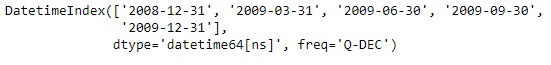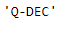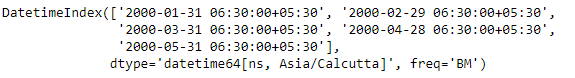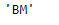# Python | Pandas DatetimeIndex.inferred_freq

• Last Updated : 24 Dec, 2018

Python is a great language for doing data analysis, primarily because of the fantastic ecosystem of data-centric python packages. Pandas is one of those packages and makes importing and analyzing data much easier.

Pandas` DatetimeIndex.inferred_freq` attribute tries to return a string representing a frequency guess, generated by infer_freq. For those cases in which the function is not able to auto detect the frequency of the DatetimeIndex it returns None.

Attention geek! Strengthen your foundations with the Python Programming Foundation Course and learn the basics.

To begin with, your interview preparations Enhance your Data Structures concepts with the Python DS Course. And to begin with your Machine Learning Journey, join the Machine Learning - Basic Level Course

Syntax: DatetimeIndex.inferred_freq

Return: freq

Example #1: Use `DatetimeIndex.inferred_freq` attribute to auto detect the frequency of the given DatetimeIndex object.

 `# importing pandas as pd``import` `pandas as pd`` ` `# Create the DatetimeIndex``didx ``=` `pd.date_range(``"2008-12-30"``, periods ``=` `5``, freq ``=``'Q'``)`` ` `# Print the DatetimeIndex``print``(didx)`

Output :Now we want the function to auto detect the frequency of the given DatetimeIndex object.

 `# find the frequency of the object.``didx.inferred_freq`

Output :As we can see in the output, the function has tried to auto detect the frequency of the given DatetimeIndex object and has returned a quarter type frequency starting from the month of December.

Example #2: Use `DatetimeIndex.inferred_freq` attribute to auto detect the frequency of the given DatetimeIndex object.

 `# importing pandas as pd``import` `pandas as pd`` ` `# Create the DatetimeIndex``didx ``=` `pd.DatetimeIndex(start ``=``'2000-01-31 06:30'``, freq ``=``'BM'``, ``                           ``periods ``=` `5``, tz ``=``'Asia/Calcutta'``)`` ` `# Print the DatetimeIndex``print``(didx)`

Output :Now we want the function to auto detect the frequency of the given DatetimeIndex object.

 `# find the frequency of the object.``didx.inferred_freq`

Output :As we can see in the output, the function has tried to auto detect the frequency of the given DatetimeIndex object and has returned ‘BM’ (Business Month end) frequency.

My Personal Notes arrow_drop_up